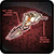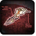Conditions

## Related effects, buffs and debuffs

Please click on an effect below to view its details.

• [does not expire]Locuste Adno
Vitesse de déplacement augmentée.

 Slot: Buff Duration: passive Tick rate: does not tick # occurrences: 0 Tags: tag.​abl.​player.​mount_ability, tag.​abl.​player.​mount
• On Apply

Only when the following conditions are met:

Perform the following actions:

• Summon a mount
• Custom
- Param 4 = (string)
- Param 5 = (string)
- Param 6 = (string)
- Param 7 = (string)
- Param 8 = (string)
- Param 9 = (string)
- Param 1 = (int) 6855115466292073041
- Param 2 = (int) 16140956924162549398
- Param 3 = (int) 2470735036708605092
• On Apply

Only when the following conditions are met:

Perform the following actions:

• Summon a mount
• Custom
- Param 4 = (string)
- Param 5 = (string)
- Param 6 = (string)
- Param 7 = (string)
- Param 8 = (string)
- Param 9 = (string)
- Param 1 = (int) 6855115466292073041
- Param 2 = (int) 16141170602253920339
- Param 3 = (int) 2470735036708605092
• On Apply

Only when the following conditions are met:

Perform the following actions:

• Summon a mount
• Custom
- Param 4 = (string)
- Param 5 = (string)
- Param 6 = (string)
- Param 7 = (string)
- Param 8 = (string)
- Param 9 = (string)
- Param 1 = (int) 6855115466292073041
- Param 2 = (int) 16140968904671250529
- Param 3 = (int) 2470735036708605092
• Just before this effect is removed

Perform the following actions:Effect #2